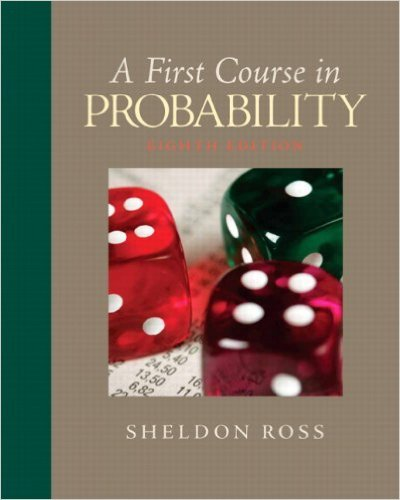×
Get Full Access to First Course In Probability - 8 Edition - Chapter 5 - Problem 5.23
Get Full Access to First Course In Probability - 8 Edition - Chapter 5 - Problem 5.23

×

# One thousand independent rolls of a fair die willbe made.ISBN: 9780136033134 163

## Solution for problem 5.23 Chapter 5

First Course in Probability | 8th Edition

• Textbook Solutions
• 2901 Step-by-step solutions solved by professors and subject experts
• Get 24/7 help from StudySoup virtual teaching assistantsFirst Course in Probability | 8th Edition

4 5 1 319 Reviews
20
3
Problem 5.23

One thousand independent rolls of a fair die willbe made. Compute an approximation to the probabilitythat the number 6 will appear between 150and 200 times inclusively. If the number 6 appearsexactly 200 times, find the probability that thenumber 5 will appear less than 150 times.

Step-by-Step Solution:
Step 1 of 3

Step 2 of 3

Step 3 of 3

##### ISBN: 9780136033134

The full step-by-step solution to problem: 5.23 from chapter: 5 was answered by , our top Statistics solution expert on 11/23/17, 05:06AM. This full solution covers the following key subjects: times, Probability, appear, compute, die. This expansive textbook survival guide covers 10 chapters, and 495 solutions. First Course in Probability was written by and is associated to the ISBN: 9780136033134. This textbook survival guide was created for the textbook: First Course in Probability, edition: 8. The answer to “One thousand independent rolls of a fair die willbe made. Compute an approximation to the probabilitythat the number 6 will appear between 150and 200 times inclusively. If the number 6 appearsexactly 200 times, find the probability that thenumber 5 will appear less than 150 times.” is broken down into a number of easy to follow steps, and 45 words. Since the solution to 5.23 from 5 chapter was answered, more than 236 students have viewed the full step-by-step answer.

Unlock Textbook Solution